﻿ GATE CS Applied Course

## GATE Previous Subject Wise Questions

##### Question 121
Consider a binary tree T that has 200 leaf nodes. Then, the number of nodes in T that have exactly
two children are ________.
Submit
Data Structures GATE 2015 SET3 Binary-Trees
##### Question 122
Given a hash table T with 25 slots that stores 2000 elements, the load factor α  for T  is
____________.
Submit
Data Structures GATE 2015 SET3 Hashing
##### Question 123
Consider the following relation

Which of the following options will be needed at the end of the SQL query
FROM Cinema P1

such that it always finds the addresses of theaters with maximum capacity?
A
WHERE P1.capacity >= All (select P2.capacity from Cinema P2)
B
WHERE P1.capacity >= Any (select P2.capacity from Cinema P2)
C
WHERE P1.capacity > All (select max(P2.capacity) from Cinema P2)
D
WHERE P1.capacity > Any (select max(P2.capacity) from Cinema P2)
GATE 2015 SET3
##### Question 124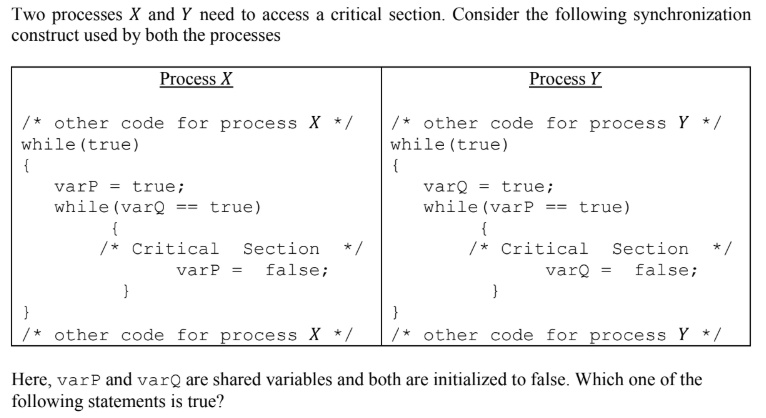A
The proposed solution prevents deadlock but fails to guarantee mutual exclusion
B
The proposed solution guarantees mutual exclusion but fails to prevent deadlock
C
The proposed solution guarantees mutual exclusion and prevents deadlock
D
The proposed solution fails to prevent deadlock and fails to guarantee mutual exclusion
operating systems GATE 2015 SET3
##### Question 125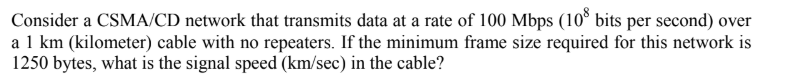A
8000
B
10000
C
16000
D
20000
Computer Networks GATE 2015 SET3
##### Question 126
Consider the following policies for preventing deadlock in a system with mutually exclusive
resources.

I. Processes should acquire all their resources at the beginning of execution. If any resource is
not available, all resources acquired so far are released
II. The resources are numbered uniquely, and processes are allowed to request for resources
only in increasing resource numbers
III. The resources are numbered uniquely, and processes are allowed to request for resources
only in decreasing resource numbers
IV. The resources are numbered uniquely. A process is allowed to request only for a resource
with resource number larger than its currently held resources

Which of the above policies can be used for preventing deadlock?
A
Any one of I and III but not II or IV
B
Any one of I, III, and IV but not II
C
Any one of II and III but not I or IV
D
Any one of I, II, III, and IV
operating systems GATE 2015 SET3
##### Question 127
In the network 200.10.11.144/27, the fourth octet (in decimal) of the last IP address of the network
which can be assigned to a host is ____________.
Submit
Computer Networks GATE 2015 SET3
##### Question 128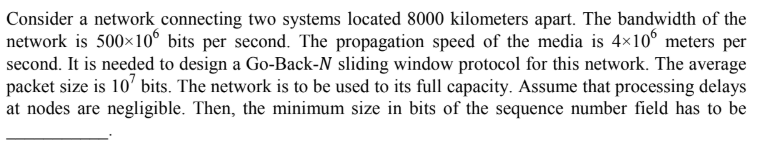Submit
Computer Networks GATE 2015 SET3
##### Question 129A
Only S1 is true
B
Only S2 is true
C
Only S1 and S3 are true
D
Only S2 and S3 are true
computer organization GATE 2015 SET3
##### Question 130A
First Come First Serve
B
Non-preemptive Shortest Job First
C
Shortest Remaining Time
D
Round Robin with Quantum value two
operating systems GATE 2015 SET3
##### Question 131
Consider the statement
“Not all that glitters is gold”
Predicate glitters(x) is true if x glitters and predicate gold(x) is true if x is gold. Which one of the following logical formulae represents the  above statement?

AB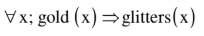C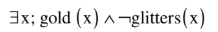D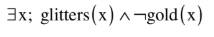GATE 2014 SET 1
##### Question 132
Let G=(V,E) be a directed graph where V is the set of vertices and E the set of edges. Then which one of the following graphs has the same strongly connected components as G ?
ABCD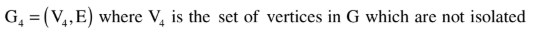GATE 2014 SET 1
##### Question 133
Let G be a graph with n vertices and m edges. What is the tightest upper bound on the running time on Depth First Search of G? Assume that the graph is represented using adjacency matrix.
A
θ(n)
B
θ(n+m)
C
θ(n2 )
D
θ(m2 )
Data Structures GATE 2014 SET 1 Graphs
##### Question 134
Consider a rooted Binary tree represented using pointers. The best upper bound on the time required to determine the number of subtrees having having exactly 4 nodes O(na Logn b). Then the value of a + 10b is ________
A
1
B
2
C
3
D
4
Data Structures GATE 2014 SET 1 Time-Complexity
##### Question 135
Consider the directed graph given below.Which one of the following is TRUE?
A
The graph does not have any topological ordering
B
Both PQRS and SRQP are topological orderings
C
Both PSRQ and SPRQ are topological orderings.
D
PSRQ is the only topological ordering.
Data Structures GATE 2014 SET 1 Graphs
##### Question 136
Let P be a QuickSort Program to sort numbers in ascending order using the first element as pivot. Let t1 and t2 be the number of comparisons made by P for the inputs {1, 2, 3, 4, 5} and {4, 1, 5, 3, 2} respectively. Which one of the following holds?
A
t1 = 5
B
t1 < t2
C
t1 > t2
D
t1 = t2
Data Structures GATE 2014 SET 1 Quick-Sort
##### Question 137
A machine has a 32-bit architecture, with 1-word long instructions. It has 64 registers, each of
which is 32 bits long. It needs to support 45 instructions, which have an immediate operand
in addition to two register operands. Assuming that the immediate operand is an unsigned
integer, the maximum value of the immediate operand is ____________.
Submit
computer organization GATE 2014 SET 1
##### Question 138
Suppose a disk has 201 cylinders, numbered from 0 to 200. At some time the disk arm is at cylinder 100, and there is a queue of disk access requests for cylinders 30, 85, 90, 100, 105, 110, 135 and 145. If Shortest-Seek Time First (SSTF) is being used for scheduling the disk access, the request for cylinder 90 is serviced after servicing ____________ number of requests.
Submit
operating systems GATE 2014 SET 1
##### Question 139
Which one of the following is FALSE?
A
User level threads are not scheduled by the kernel.
B
When a user level thread is blocked, all other threads of its process are blocked.
C
Context switching between user level threads is faster than context switching between kernel level threads.
D
Kernel level threads cannot share the code segment
operating systems GATE 2014 SET 1
##### Question 140
Given the following statements:
    S1: A foreign key declaration can always
be replaced by an equivalent check
assertion in SQL.
S2: Given the table R(a,b,c) where a and
b together form the primary key, the
following is a valid table definition.
CREATE TABLE S (
a INTEGER,
d INTEGER,
e INTEGER,
PRIMARY KEY (d),
FOREIGN KEY (a) references R) 
Which one of the following statements is CORRECT?
A
S1 is TRUE and S2 is FALSE.
B
Both S1 and S2 are TRUE.
C
S1 is FALSE and S2 is TRUE.
D
Both S1 and S2 are FALSE.
GATE 2014 SET 1© 2020 - All rights are reserved- AAIC Technologies pvt ltd